cancel
Showing results for
Did you mean:

Fabric is Generally Available. Browse Fabric Presentations. Work towards your Fabric certification with the Cloud Skills Challenge.Regular Visitor

## Removal of blanks from sub row in matrix while maintaining total value

Hello community,

Need your help with a following scenario.

I have a data table set where there are blanks in certain columns.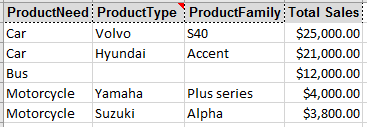When I create a matrix value, I get the below table with blank rows being displayed in the product type and Product family for Bus Product need categoryI want to display the all categories and restrict the blank row display for Product Type and Product Family and still be able to show the complete total value. If I apply filter to remove the blank from Product Type or Product family, it removes bus option completely from Product Need and the total value is also reduced. Any idea how I can limit the blank row in subsequent cateogirs without affecting total value or display on the matrix?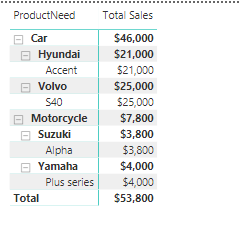1 ACCEPTED SOLUTIONSolution Sage

Re: need to create multiple vs. one formula:
The measure in #4, which I believe provides the output you are looking for, references the measures in #2 and #3. So, I presented it such that, yes, you would need to do multiple formulas to get it working. It can be done in one measure, though. See below.

First, I'll note the formula under #1 is for a calculated column, so to add it to your model, you need to go to the data layer, Modeling tab, and click the New Column button in order to add it; another way would be to right click on the table in data model and click "New column"

If you create the calculated column in the model, it's relatively straightforward to combine the three measures I provided into one if you wanted:

```TotalSalesOneMeasure =
VAR FilterDepth =
ISFILTERED(Products[ProductNeed])
+ISFILTERED(Products[ProductType])
+ISFILTERED(Products[ProductFamily])
VAR MaxDepth =
MAX(Products[HierarchyDepth])
RETURN
IF(
FilterDepth>MaxDepth,
BLANK(),
SUM(Products[Total Sales])
)```

If you really want to just put it all in one measure and not bother with a calculated column (this is not as efficient, but it's probably not noticable unless you are building complex measures off of this and/or working with larger datasets):

```TotalSalesOneMeasureNoCalcCol =
VAR FilterDepth =
ISFILTERED(Products[ProductNeed])
+ISFILTERED(Products[ProductType])
+ISFILTERED(Products[ProductFamily])
VAR MaxDepth =
MAXX(
Products,
ISTEXT([ProductNeed])
+ISTEXT([ProductType])
+ISTEXT([ProductFamily])
)
RETURN
IF(
FilterDepth>MaxDepth,
BLANK(),
SUM(Products[Total Sales])
)```

Re: error
My primary guess is that you tried to do the formula in #1 as a measure instead of a calculated column (as that is exactly the error you'd get if you tried to do that). Otherwise, it may be due to a difference between the generalized dataset you provided and your actual dataset (assuming they are different). Or it could simply be a typo-type kind of error - perhaps check the calculated column and measures to see if there are unrecognized references, etc. (although the error doesn't really imply that is the problem).

Hope that helps!

11 REPLIES 11Super User

Hi,

A much simpler way would be to replace Blanks with Unknown in the Query Editor.  The end result would be this:Regards,
Ashish Mathur
http://www.ashishmathur.comRegular Visitor

Hi Ashish_Mathur,

No, that won't work - if there is no values in the sub hiearchy, I don't want to display unknown.

I only want to limit the sub hiearchy if there is a blank.Solution Sage

One technique I use in situations like this is the following:

1. Calculate the hierarchy depth for each row in your table (i.e. calculated column)
note this assumes the blank cells are actually blank/null and not empty text ("") - if they are empty text, either make null in PowerQuery or use a different check ( e.g. LEN([column])>0 😞
`HierarchyDepth = ISTEXT([ProductNeed])+ISTEXT([ProductType])+ISTEXT([ProductFamily])`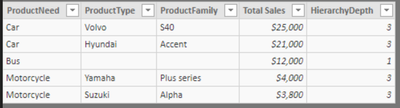2. Create a measure that calculates the filter depth
`FilterDepth = ISFILTERED(Products[ProductNeed])+ISFILTERED(Products[ProductType])+ISFILTERED(Products[ProductFamily])`3. Create a measure that calculates max depth per hierarchy
`MaxDepth = MAX(Products[HierarchyDepth])`4. Now you can wrap any measure you want in "if the filter depth is greater than the max depth of the current hierarch, then show as blank, otherwise do the measure" - specifically, for your answer
```TotalSales =
IF(
[FilterDepth]>[MaxDepth],
BLANK(),
SUM(Products[Total Sales])
)```Credit to SQLBI, as the above is a stripped down interpretation of their Parent-Child Hierarchies pattern.Regular Visitor

Hi MarkLaf,

Thanks for the note. Would I need to create three seperate DAX formulas you mentioned or it would all be under one single formula?Regular Visitor

Having another challenge, when I entered the DAX formula, getting following error message:

"A single value for column ProductNeed in table cannot be determined. This can happen when a measure formula refers to a column that contains many values"

Am I doing something incorrect?Solution Sage

Re: need to create multiple vs. one formula:
The measure in #4, which I believe provides the output you are looking for, references the measures in #2 and #3. So, I presented it such that, yes, you would need to do multiple formulas to get it working. It can be done in one measure, though. See below.

First, I'll note the formula under #1 is for a calculated column, so to add it to your model, you need to go to the data layer, Modeling tab, and click the New Column button in order to add it; another way would be to right click on the table in data model and click "New column"

If you create the calculated column in the model, it's relatively straightforward to combine the three measures I provided into one if you wanted:

```TotalSalesOneMeasure =
VAR FilterDepth =
ISFILTERED(Products[ProductNeed])
+ISFILTERED(Products[ProductType])
+ISFILTERED(Products[ProductFamily])
VAR MaxDepth =
MAX(Products[HierarchyDepth])
RETURN
IF(
FilterDepth>MaxDepth,
BLANK(),
SUM(Products[Total Sales])
)```

If you really want to just put it all in one measure and not bother with a calculated column (this is not as efficient, but it's probably not noticable unless you are building complex measures off of this and/or working with larger datasets):

```TotalSalesOneMeasureNoCalcCol =
VAR FilterDepth =
ISFILTERED(Products[ProductNeed])
+ISFILTERED(Products[ProductType])
+ISFILTERED(Products[ProductFamily])
VAR MaxDepth =
MAXX(
Products,
ISTEXT([ProductNeed])
+ISTEXT([ProductType])
+ISTEXT([ProductFamily])
)
RETURN
IF(
FilterDepth>MaxDepth,
BLANK(),
SUM(Products[Total Sales])
)```

Re: error
My primary guess is that you tried to do the formula in #1 as a measure instead of a calculated column (as that is exactly the error you'd get if you tried to do that). Otherwise, it may be due to a difference between the generalized dataset you provided and your actual dataset (assuming they are different). Or it could simply be a typo-type kind of error - perhaps check the calculated column and measures to see if there are unrecognized references, etc. (although the error doesn't really imply that is the problem).

Hope that helps!Regular Visitor

Hi MarkLaf,

Thanks for your note - I had made an error. For the HiearchyDepth, I had indeed taken it as a new measure than column, hence it wasn't working.

Question: If I am adding multiple columns for value, wouid I need to define for all those columns? In the example we had TotalSalesSolution Sage

I'm not sure I understand your question, although I attempted an answer below. If it does not address your issue, an example (additional sample data and expected output) of what you want may help.

If you mean that there are other columns in your actual dataset that you either

1. want to include in separate measures (e.g. total operating costs in addition to total sales), then simply put your desired measure within the "if the filter depth is greater than the max depth of the current hierarch, then show as blank, otherwise do the measure" logic I referenced in my initial answer. Example:
```TotalOpEx =
IF(
[FilterDepth]>[MaxDepth],
BLANK(),
SUM(Products[Total Op Ex])
)```
2. OR if you need to sum/multiply/etc. (e.g. units * unit cost) of multiple columns in your table and then show in a visual, then probably the simplest approach is to add a calculated column in the table in your data model that does said addition/multiplication/etc. that you can then reference in a measure with the same "blank if filter depth is greater than hierarchy" logic. Exmaple:
```//Calculated column on table in your model
CalcSale =
[Price] * [Quantity]```
```//Measure to use in a visual that is referencing calculated column
TotalCalcSales =
IF(
[FilterDepth]>[MaxDepth],
BLANK(),
SUM(Products[CalcSale])
)```

In both instances of example code above, I'm referencing the FilterDepth and MaxDepth measures that I described in #2 and #3 of my first response. Copying here again for ease of reference:

```FilterDepth =
ISFILTERED(Products[ProductNeed])
+ISFILTERED(Products[ProductType])
+ISFILTERED(Products[ProductFamily])

//As before, this is referencing the HierarchyDepth calculated column
MaxDepth =
MAX(Products[HierarchyDepth])```Regular Visitor

Hi MarkLaf,

Apologies if my query was a bit unclear.

Rephrasing it - with below example. In the below table for examply, if I multiple values to display in my matrix for e.g. total sales, number of units sold and ASP, would I need to add all of them in the formula like below:

```TotalSales =
IF(
[FilterDepth]>[MaxDepth],
BLANK(),
SUM(Products[Total Sales])    SUM(Products[Number of Units sold])    SUM(Products[ASP])
)```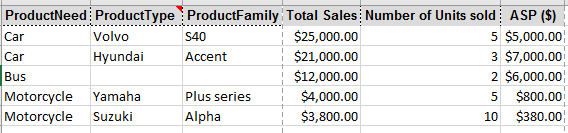Solution Sage

If all those columns are in your table in the data model, and you want to show them all in a matrix but hiding the blank row headers, then you would need to do a measure for each one.

```TotalSales =
IF(
[FilterDepth]>[MaxDepth],
BLANK(),
SUM(Products[Total Sales])
)```
```TotalUnits =
IF(
[FilterDepth]>[MaxDepth],
BLANK(),
SUM(Products[Number of Units sold])
)```

Note that I'm actually calculating ASP in the measure rather than using an average from a calculated column in the table, as that will ensure it is correct regardless of filter context (e.g. correct for Car at summary level and for an individual product family).

```TotalASP =
IF(
[FilterDepth]>[MaxDepth],
BLANK(),
DIVIDE([TotalSales],[TotalUnits])
)```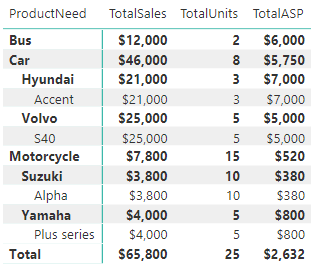Anonymous
Not applicable

I have tried this solution but I have a problem.

I have my Account Hierarchy structure in a seperate table, connected to the fact table using a bridge.

My FilterDebth is always max, I dont know how to get it to give me the correct number when I am expanding my account hierarchy in my P&L statement. I use the matrix visualization.

My formulas:

As expression:

FilterDepth2 =
ISFILTERED(AccountHierarchyFlash[Level 1 + Name])
+ISFILTERED(AccountHierarchyFlash[Level 2 + Name])
+ISFILTERED(AccountHierarchyFlash[Level 3 + Name])
+ISFILTERED(AccountHierarchyFlash[Level 4 + Name])
+ISFILTERED(AccountHierarchyFlash[Level 5 + Name])
+ISFILTERED(AccountHierarchyFlash[Level 6 + Name])
+ISFILTERED(AccountHierarchyFlash[Level 7 + Name])

As measure - correct one:
MaxDepth =
MAX(AccountHierarchyFlash[HierarchyDepth])

Inside my Account table - correct:
As calulated calumn:
HierarchyDepth =
ISTEXT([Level 1 + Name])
+ISTEXT([Level 2 + Name])
+ISTEXT([Level 3 + Name])
+ISTEXT([Level 4 + Name])
+ISTEXT([Level 5 + Name])
+ISTEXT([Level 6 + Name])
+ISTEXT([Level 7 + Name])Why is my filterdepth always 7 ???Announcements#### Power BI Monthly Update - November 2023

Check out the November 2023 Power BI update to learn about new features.#### Fabric Community News unified experience

Read the latest Fabric Community announcements, including updates on Power BI, Synapse, Data Factory and Data Activator.#### The largest Power BI and Fabric virtual conference

130+ sessions, 130+ speakers, Product managers, MVPs, and experts. All about Power BI and Fabric. Attend online or watch the recordings.Top Solution Authors
Top Kudoed Authors
Users online (3,700)